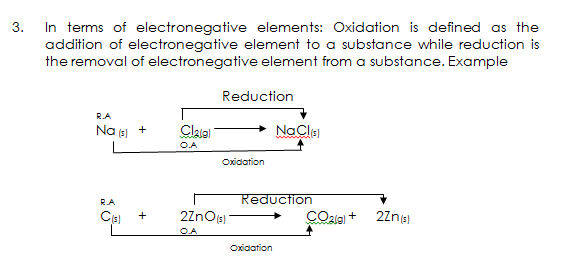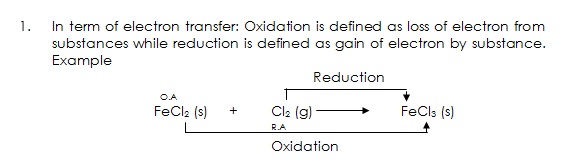# Types of Reaction/Redox Reactions

Welcome to Class !!

In today’s Chemistry class, We will be learning about the Different Types of Reactions / Redox Reactions. We hope you enjoy the class!

CONTENT

• Definition of Oxidation and Reduction.
• Relationship between Oxidation number and IUPAC naming#### TYPES OF REACTIONS

Chemical reactions are changes in which some new chemical substances are formed. Chemical reactions can be grouped into:

1. Combination reaction: This involves the combination of two or more substances to form a single compound. Example

PbO2(s)  +  SO2(g)→ PbSO4(s)

1. Displacement reaction: In displacement reaction, one element or radical replaces another element or radical in a compound. Example

Zn(s)  +  CuSO4(aq)→ ZnSO4(aq)  +  Cu(s)

1. Double decomposition: This reaction involves the reactants decomposing to form new substances by an exchange of radicals. Example

AgNO3(aq)  +  NaCl(aq) →AgCl(s)  +  NaNO3(aq)

1. Reversible reaction: A reversible reaction is a reaction which the products can react to give the reactants. Example

CaCO3(s)  +  H2O(l)  +  CO2(g)  →Ca(HCO3)2(aq)

1. Thermal dissociation: In thermal dissociation, each molecule of a substance dissociates into two simpler molecules or atoms on the application of heat. Unlike thermal decomposition, thermal dissociation is reversible. Example

NH4Cl(s)          → NH3(g)  +  HCl(g)

1. Catalytic reaction: Any chemical reaction which uses a catalyst is a catalytic reaction. E.g.

H2(g)  +  I2(g) → 2HI(g)

A catalyst is a substance which alters the rate of a chemical reaction, but itself remains chemically and quantitatively unchanged at the end of the reaction.

#### CHARACTERISTICS OF A CATALYST

1. It alters the rate of a chemical reaction.
2. It remains unchanged in chemical nature and mass at the end of a reaction.
3. It is specific in action.
4. It cannot start a reaction.
5. It does not affect the types of products formed in a reaction.
6. It has no effect on the equilibrium of a reversible reaction.
7. The effect of a solid catalyst is improved by increasing its surface area.

TYPES OF CATALYSTS

Catalysts may be inorganic or organic substances. Inorganic catalysts can be grouped into positive and negative catalysts. Organic catalysts are known as enzymes and are produced by living cells.

A catalyst which increases the rate of a reaction is a positive catalyst. An example is manganese (IV) oxide which speeds up the decomposition of potassium trioxochlorate (V).

2KClO3(s)→2KCl(s)  +  3O2(g)

A catalyst which decreases the rate of a reaction is a negative catalyst. Example, tetraoxophosphate (V) acid decreases the rate of decomposition of hydrogen peroxide.

2H2O2(l)→ 2H2O(l)  +  O2(g)

Promoters and Inhibitors: A promoter is a substance that influences the rate of a reaction by affecting the efficiency of the catalyst. Example, aluminium oxide is added to an iron catalyst used in the Haber process for the production of ammonia.

An inhibitor is a substance that decreases the efficiency of a catalyst. Example, hydrogen cyanide and mercury salts inhibit important enzymes in our bodies.

#### TYPES OF CATALYSIS

Homogeneous catalysis: In this reaction, the catalyst, reactants and products are all in the same phase. Example

2SO2(g)  +  O2(g) → 2SO3(g)

Heterogeneous catalysis: For heterogeneous catalysis, the reactants, products and catalysts are in different phases. Example

H2(g)  +  I2(g) → 2HI(g)

EVALUATION

1. State what type of reaction is represented by each of the following equations:
• 2KBr(aq) +  Cl2(g)→ 2KCl(aq)  +  Br2(l)
• 2Pb(NO3)2(s)→ 2PbO(s) +  4NO2(g)  +  O2(g)
• KNO3(aq) +  H2SO4(aq)→ KHSO4(aq)
2. Define the term Catalyst and give five characteristics of a catalyst.

### OXIDATION AND REDUCTION

DEFINITION OF OXIDATION AND REDUCTION

The following definitions have been used for the oxidation and reduction processes

• In terms of Oxygen: Oxidation is defined as the addition of oxygen to a substance while reduction is defined as the removal of oxygen from a substance. Examples

Reduction

2Mg(s)+    O2(g)                     2MgO(s)

R.A        O.A

Oxidation

R.A Reduction

C(s)      +          2ZnO(s)                              CO2(s)   + 2Zn(s)

O.A

Oxidation

• In terms of Hydrogen: Oxidation is defined as the removal of hydrogen from a substance while reduction is the addition of hydrogen to a substance. Example

Reduction

R.A

H2S(g)     +        Cl2(g)                      2HCl(g)    +          S(s)

O.A

Oxidation

• In terms of electronegative elements: Oxidation is defined as the addition of an electronegative element to a substance while reduction is the removal of an electronegative element from a substance. Example

Reduction

R.A

Na (s)   +          Cl2(g)                  NaCl(s)

O.A

Oxidation

R.A                                    Reduction

C(s)      +          2ZnO(s)                       CO2(g)            +     2Zn(s)

O.A

Oxidation• In term of electron transfer: Oxidation is defined as loss of an electron from substances while reduction is defined as a gain of electrons by a substance. Example

Reduction

O.A

FeCl2 (s)         +          Cl2 (g)                        FeCl3 (s)

R.A

OxidationEVALUATION

1. Define oxidation and reduction in terms of
• (a) Hydrogen (b) Oxygen
1. Give the modern definition of Oxidation and Reduction

#### RELATIONSHIP BETWEEN OXIDATION NUMBER AND IUPAC NAMING

The oxidation number or state of an element in a neutral compound or radical (ion) is defined as the numerical electrical charge it appears to have as determined by a set of arbitrary rules.­

RULES OF DETERMINING OXIDATION NUMBERS

1. The oxidation number of all uncombined element is zero e.g. Na, Cl
2. The oxidation number of an ion consisting of a single element is the same as the ionic charge on it. E.g. oxidation number of Na+ is +1, Cl is -1
3. The oxidation number of an ion consisting of more than one element (radical) is the algebraic sum of the oxidation number of all the elements in the ion.

Example for OH

[Oxidation no. of O]        +     [oxidation no. of H]       =      [oxidation no of OH]

(-2)                    +                     (+1)                       =                   -1

The oxidation number of NH4+   is +1,

1. The oxidation number of a neutral compound is zero and it is the algebraic sum of the oxidation number of all the elements in the compound.

Example: MgCl2= 0

[Oxidation no. of Mg]   + 2[oxidation of no. Cl] = [oxidation number of MgCl2]

+2                                  +           2 x (-1)            =                  +2 -2         =      0

EXAMPLE

1. Find the oxidation number of the manganese atom in KMnO4 and give the IUPAC name the compound.

Solution:

KMnO4 = 0

(+1) + Mn + 4(-2) =0

+1 +Mn -8=0

Mn – 7 =0

Mn = +7

The oxidation number (state) of manganese in KMnO4 is + 7.

Hence the IUPAC name of the compound is Potassium tetraoxomanganate (VII)

1. Find the oxidation number of chlorine in NaClO and give the IUPAC name of the compound.

Solution:

NaClO = 0

(+1) + Cl + (-2) = 0

Cl -2 + 1 = 0

Cl -1  = 0

Cl = +1

The oxidation number of chlorine in NaClO is +1.

Thus, the IUPAC name of the compound is Sodium oxochlorate (I)

#### IDENTIFYING REDOX REACTIONS USING OXIDATION NUMBER

Redox reactions can be identified with the aid of oxidation number. For a redox reaction, the oxidation number of an element on the reactant side is different on the product side. There must be changes in oxidation numbers.

Example:      C   +   ZnO                 CO2   +          Zn                   Redox

0         +2                  +4               0

NaOH   +         HCl                     NaCl        +          H2O                Not Redox

(+1)(-2)(+1)     (+1)(-1)             (+1)(-1)           (+1×2) (-2)

### GENERAL EVALUATION/REVISION

1. Show that the following reactions are redox reaction
• 2H2S(g) +  SO2(g)→3S(s)  +  2H2O(l)
• 2K(s) +  2H2O(l)→ 2KOH(aq)  +  H2(g)
• 2FeCl(aq) +  Cl2(g)→ 2FeCl3(aq)
1. What is the oxidation number of chromium in K2Cr2O7? Give the IUPAC name of the compound.
2. Define oxidation and reduction in modern terms
3. Define radicals and give TWO examples
4. What is valency? Give the valency of the following elements: sodium, phosphorus, carbon, sulphur, and aluminium.

READING ASSIGNMENT: New School Secondary for Senior Secondary School by O. Y. Ababio, pages 184-192

WEEKEND ASSIGNMENT

SECTION A: Write the correct option ONLY

1. A major difference between thermal dissociation and thermal decomposition is that
2. Thermal dissociation is not A. reversible B. thermal decomposition is reversible C. thermal dissociation is reversible D. thermal decomposition is not reversible.
3. Removal of hydrogen from a substance is A. oxidation B. reduction C. oxidizing agent D. reducing agent
4. The oxidation number of an uncombined element is A. 2 B. 4 C. –1 D. 0.
5. H2S +  O2                        S + H2O

In the above equation of reaction, the oxidation number of sulphur changes from

A. –2 to 0 B. +2 to –1 C. 0 to +2 D. +1 to -2

6. What is the oxidation number of chromium in sodium heptaoxodichromate (VI)? A. +3  B. +12  C. +6  D. +2

SECTION B

1. Define oxidation and reduction in terms of electron transfer.
2. Determine the oxidation number of Chromium in K2Cr2O7.

We have come to the end of this class. We do hope you enjoyed the class?

Should you have any further question, feel free to ask in the comment section below and trust us to respond as soon as possible.

In our next class, we will be learning about Oxidizing and Reducing Agents. We are very much eager to meet you there.

Are you a Parent? Share your quick opinion and win free 2-month Premium Subscription

Don`t copy text!